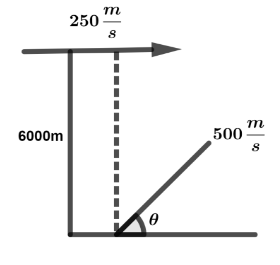Courses
Courses for Kids
Free study material
Offline Centres
MoreLast updated date: 05th Dec 2023
Total views: 281.4k
Views today: 8.81k

# An aircraft moving with a speed of $250\,m{s^{ - 1}}$at a height of, overhead by anti aircraft-gun. If the muzzle velocity is $500\,m{s^{ - 1}}$, the firing angle $\theta$ should be:A. ${30^ \circ }$B. ${60^ \circ }$C. ${45^ \circ }$D. ${75^ \circ }$Verified
281.4k+ views
Hint:From the given question we have to find out the angle at a certain point for this type of calculation we are going to use the given data and trigonometry formulas. Before going into the solution part once check the given values.

When an aircraft is moving with a speed of $250\,m{s^{ - 1}}$at a height of $6000\,m$. From ground we are hiring an anti aircraft-gun with speed $500\,m{s^{ - 1}}$with an angle $\theta$.Now we are assuming the horizontal component and vertical component, whereas horizontal component is $500\cos \theta$ and vertical component is $500\sin \theta$.
For overhead an anti aircraft gun $u\left( x \right) = 250m{s^{ - 1}} \to \left( 1 \right)$
Given that $u\left( x \right) = 500\cos \theta$
Substitute the value of $u\left( x \right)$in equation (1), then we get
$500\cos \theta = 250 \\ \Rightarrow \cos \theta = \dfrac{{250}}{{500}} \\ \Rightarrow \cos \theta = \dfrac{1}{2} \\ \Rightarrow \theta = {\cos ^{ - 1}}\dfrac{1}{2} \\$
From trigonometry formulas we know the values of ${\cos ^{ - 1}}\theta$ where as ${\cos ^{ - 1}}\dfrac{1}{2}$ that means the $\theta$ value is, $\theta = {60^ \circ }$.

Hence, the correct option is B.